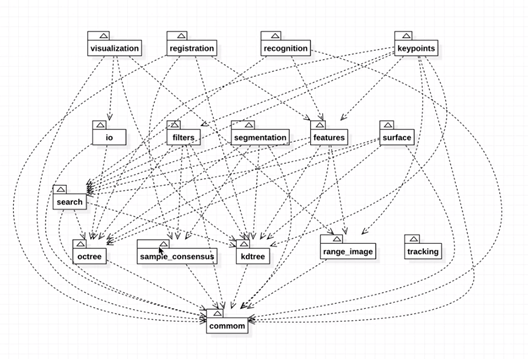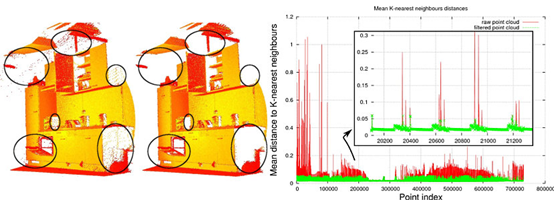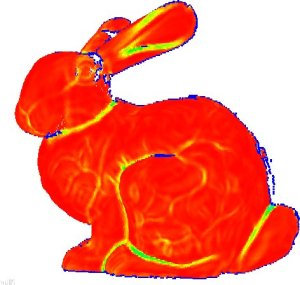31

2021

## 一．初识PCL

This tutorials will walk you through the components of your PCL installation, providing short descriptions of the modules, indicating where they are located and also listing the interaction between different components.## 二．总览

PCL is split in a number of modular libraries. The most important set of released PCL modules is shown below:
PCL库是一个模组化的库。PCL发行库中最重要的几个组成部分如下：
Filters滤波
Features特征提取
KeyPoints关键点
Registration

## 三．滤波

Background

An example of noise removal is presented in the figure below. Due to measurement errors, certain datasets present a large number of shadow points. This complicates the estimation of local point cloud 3D features. Some of these outliers can be filtered by performing a statistical analysis on each point’s neighborhood, and trimming those that do not meet a certain criteria. The sparse outlier removal implementation in PCL is based on the computation of the distribution of point to neighbor distances in the input dataset. For each point, the mean distance from it to all its neighbors is computed. By assuming that the resulting distribution is Gaussian with a mean and a standard deviation, all points whose mean distances are outside an interval defined by the global distances mean and standard deviation can be considered as outliers and trimmed from the dataset.Documentation: http://docs.pointclouds.org/trunk/group__filters.html（科学上网）
Interacts with:
Sample Consensus
Kdtree
Octree

KD树

## 四．特征

Background

A theoretical primer explaining how features work in PCL can be found in the 3D Features tutorial.

The features library contains data structures and mechanisms for 3D feature estimation from point cloud data. 3D features are representations at certain 3D points, or positions, in space, which describe geometrical patterns based on the information available around the point. The data space selected around the query point is usually referred to as the k-neighborhood.

The following figure shows a simple example of a selected query point, and its selected k-neighborhood.

An example of two of the most widely used geometric point features are the underlying surface’s estimated curvature and normal at a query point p.

Both of them are considered local features, as they characterize a point using the information provided by its k closest point neighbors.

For determining these neighbors efficiently, the input dataset is usually split into smaller chunks using spatial decomposition techniques such as octrees or kD-trees, and then closest point searches are performed in that space.

Depending on the application one can opt for either determining a fixed number of k points in the vicinity of p, or all points which are found inside of a sphere of radius r centered at p.

Unarguably, one the easiest methods for estimating the surface normals and curvature changes at a point p is to perform an eigendecomposition (i.e., compute the eigenvectors and eigenvalues) of the k-neighborhood point surface patch.

Thus, the eigenvector corresponding to the smallest eigenvalue will approximate the surface normal n at point p, while the surface curvature change will be estimated from the eigenvalues as with .

λ0/(λ0+λ1+λ2 )其中(λ0<λ1<λ2)……………………（公式1-1）Documentation: http://docs.pointclouds.org/trunk/group__features.html
Interacts with:
Common
Search
KdTree
Octree
Range Image

KD树

Written By Epoch_kiwiii

TAG